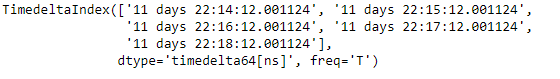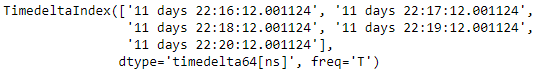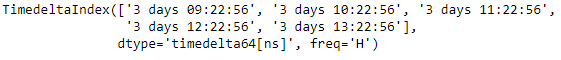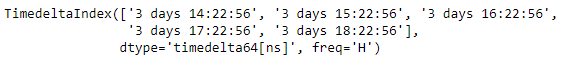Related Articles

# Python | Pandas TimedeltaIndex.shift()

• Last Updated : 01 Jan, 2019

Python is a great language for doing data analysis, primarily because of the fantastic ecosystem of data-centric python packages. Pandas is one of those packages and makes importing and analyzing data much easier.

Pandas` TimedeltaIndex.shift()` function performs specialized shift on the given TimedeltaIndex object, which produces a DatetimeIndex object.

Syntax : TimedeltaIndex.shift(n, freq=None)

Parameters :
n : Periods to shift by
freq : DateOffset or timedelta-like, optional

Return : shifted : DatetimeIndex

Example #1: Use `TimedeltaIndex.shift()` function to shift the given TimedeltaIndex object by 2 periods.

 `# importing pandas as pd``import` `pandas as pd`` ` `# Create the TimedeltaIndex object``tidx ``=` `pd.TimedeltaIndex(start ``=``'11 days 22:14:12.001124'``,``                                   ``periods ``=` `5``, freq ``=``'T'``)`` ` `# Print the TimedeltaIndex object``print``(tidx)`

Output :Now we will use the `TimedeltaIndex.shift()` function to shift each element of the given TimedeltaIndex object by 2 periods.

 `# shift by 2 periods``tidx.shift(n ``=` `2``)`

Output :As we can see in the output, the `TimedeltaIndex.shift()` function has returned a new object and it has shifted each element by 2 minutes.

Example #2: Use `TimedeltaIndex.shift()` function to shift the given TimedeltaIndex object by 2 periods.

 `# importing pandas as pd``import` `pandas as pd`` ` `# Create the TimedeltaIndex object``tidx ``=` `pd.TimedeltaIndex(start ``=``'03 days 09:22:56'``,``                            ``periods ``=` `5``, freq ``=``'H'``)`` ` `# Print the TimedeltaIndex object``print``(tidx)`

Output :Now we will use the `TimedeltaIndex.shift()` function to shift each element of the given TimedeltaIndex object by 5 periods.

 `# shift by 5 periods``tidx.shift(n ``=` `5``)`

Output :As we can see in the output, the `TimedeltaIndex.shift()` function has returned a new object and it has shifted each element by 5 hours.

Attention geek! Strengthen your foundations with the Python Programming Foundation Course and learn the basics.

To begin with, your interview preparations Enhance your Data Structures concepts with the Python DS Course. And to begin with your Machine Learning Journey, join the Machine Learning – Basic Level Course

My Personal Notes arrow_drop_up